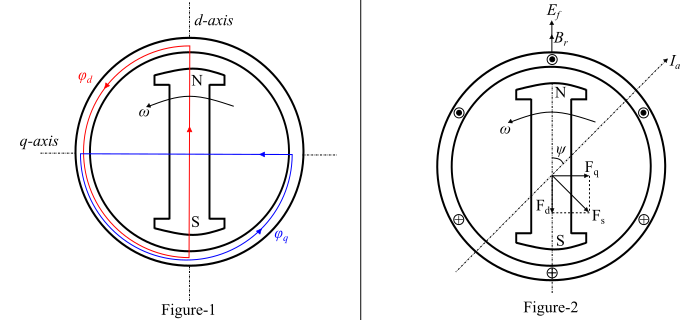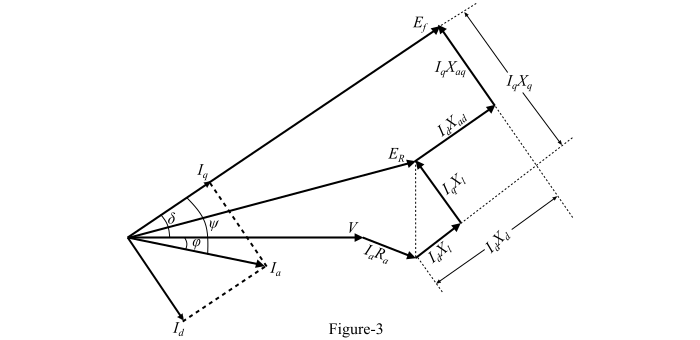# Two Reaction Theory of Salient Pole Synchronous Machine (Alternator)

In a salient-pole rotor synchronous machine, the air-gap is highly non-uniform. Consider a synchronous machine having a 2-pole salient-pole rotor rotating in the anti-clockwise direction within a 2-pole stator, as shown in Figure-1.

In Figure-1, the axis shown along the axis of the rotor is known as direct axis or d-axis and the axis perpendicular to the d-axis is called quadrature axis or q-axis. It can be seen that the two small air-gaps are involved in the path of d-axis flux ($𝜑_{𝑑}$), thus the reluctance of the path is minimum. The q-axis flux ($𝜑_{𝑞}$) path has two large air-gaps and it is the path of maximum reluctance.The rotor magnetic field ($𝑩_{𝑟}$) is shown directed vertically upward in Figure-2. This rotor magnetic field induces an EMF in the armature (or stator winding).

If a lagging power factor load is connected to the alternator, an armature current ($𝑰_{𝒂}$) will flow. The armature current ($𝑰_{𝑎}$) lags behind the excitation voltage ($𝑬_{𝑓}$) by an angle ψ (see Figure-2). The armature current ($𝑰_{𝑎}$) produces the stator MMF ($𝐹_{𝑠}$) which lags behind $𝑰_{𝒂}$ by 90°. The stator MMF ($𝐹_{𝑠}$) produces the stator magnetic field ($𝑩_{𝒔}$) along the direction of $𝐹_{𝑠}$.

According to Blondel’s Two Reaction Theory, the stator MMF ($𝐹_{𝑠}$) can be resolved into two components viz. the direct-axis component ($𝐹_{𝑑}$) and the quadrature axis component ($𝐹_{𝑞}$).

If

• $𝜑_{𝑑}$ = Direct axis flux

• $𝜑_{𝑞}$ = Quadrature axis flux

• $𝑆_{𝑑}$ = Reluctance of direct axis flux path

• $𝑆_{𝑞}$ = Reluctance of quadrature axis flux path

Then,

$$\mathrm{Direct\:axis\:flux,\:𝜑_{𝑑} =\frac{𝐹_{𝑑}}{𝑆_{𝑑}}… (1)}$$

And,

$$\mathrm{Qaudrature\:axis\:flux,\:𝜑_{𝑞} =\frac{𝐹_{𝑞}}{𝑆_{𝑞}}… (2)}$$

Since $𝑆_{𝑑} < 𝑆_{𝑞}$ , the direct axis component ($𝐹_{𝑑}$) of stator MMF produces more flux than the quadrature axis component ($𝐹_{𝑞}$) of the stator MMF. The direct axis and quadrature axis components of the stator fluxes produce voltages in the stator winding by armature reaction.

Let,

• $𝑬_{𝑎𝑑}$ = Direct axis component of armature reaction voltage

• $𝑬_{𝑎𝑞}$ = Quadrature axis component of armature reaction voltage

Since each armature reaction voltage is directly proportional to respective armature current and lags behind the armature current by 90°, the armature reaction voltages can be written as,

$$\mathrm{𝑬_{𝑎𝑑} = −𝑗𝑰_{𝑑}𝑋_{𝑎𝑑} … (3)}$$

$$\mathrm{𝑬_{𝑎𝑞} = −𝑗𝑰_{𝑞}𝑋_{𝑎𝑞} … (4)}$$

Where,

• $𝑋_{𝑎𝑑}$ is the armature reaction reactance in the direct axis per phase.

• $𝑋_{𝑎𝑞}$ is the armature reaction reactance in the quadrature axis per phase.

Here, $𝑋_{𝑎𝑞}$ < $𝑋_{𝑎𝑑}$ because the EMF induced by a given MMF acting on the direct axis is smaller than the EMF on the quadrature axis due to its higher reluctance.

Now, the resultant EMF induced in the machine is,

$$\mathrm{𝑬_{𝑅} = 𝑬_{𝑓} + 𝑬_{𝑎𝑑 }+ 𝑬_{𝑎𝑞}}$$

$$\mathrm{\Rightarrow\:𝑬_{𝑅}=𝑬_{𝑓}-𝑗𝑰_{𝑑}𝑋_{𝑎𝑑}-𝑗𝑰_{𝑞}𝑋_{𝑎𝑞}… (5)}$$

Also, the resultant voltage ($𝑬_{𝑅}$) is equal to the phasor sum of terminal voltage and the voltage drops in the resistance and leakage reactance of the armature, thus,

$$\mathrm{𝑬_{𝑅}=𝑽 + 𝑰_{𝑎}𝑅_{𝑎} + 𝑗𝑰_{𝑎}𝑋_{𝑙 }… (6)}$$

The armature current ($𝑰_{𝑎}$) is split into two components, one in phase with the excitation voltage ($𝑬_{𝑓}$) and the other in phase quadrature to it.

If

• $𝑰_{𝑞}$ = quadrature axis component of $𝑰_{𝑎}$ in phase with $𝑬_{𝑓}$

• $𝑰_{𝑑}$ = direct axis component of $𝑰_{𝑎}$ lagging $𝑬_{𝑓}$ by 90°

Then, the total armature current is the phasor sum of $𝑰_{𝑞}$ and $𝑰_{𝑑}$, i.e.,

$$\mathrm{𝑰_{𝑎} = 𝑰_{𝑞} + 𝑰_{𝑑} … (7)}$$

Now, from eqns. (5) and (6), we get,

$$\mathrm{𝑬_{𝑓} = 𝑽 + 𝑰_{𝑎}𝑅_{𝑎} + 𝑗𝑰_{𝑎}𝑋_{𝑙} + 𝑗𝑰_{𝑑}𝑋_{𝑎𝑑} + 𝑗𝑰_{𝒒}𝑋_{𝑎𝑞} … (8)}$$

And, from Eqns. (7) and (8), we get,

$$\mathrm{𝑬_{𝑓} = 𝑽 + (𝑰_{𝑞} + 𝑰_{𝑑})𝑅_{𝑎} + 𝑗(𝑰_{𝑞} + 𝑰_{𝑑})𝑋_{𝑙} + 𝑗𝑰_{𝑑}𝑋_{𝑎𝑑} + 𝑗𝑰_{𝒒}𝑋_{𝑎𝑞}}$$

$$\mathrm{\Rightarrow\:𝑬_{𝑓 }= 𝑽 + (𝑰_{𝑞 }+ 𝑰_{𝑑})𝑅_{𝑎} + 𝑗𝑰_{𝑑}(𝑋_{𝑙} + 𝑋_{𝑎𝑑}) + 𝑗𝑰_{𝑞} (𝑋_{𝑙} + 𝑋_{𝑎𝑞} )}$$

$$\mathrm{\Rightarrow\: 𝑬_{𝑓 }= 𝑽 + (𝑰_{𝑞} + 𝑰_{𝑑})𝑅_{𝑎} + 𝑗𝑰_{𝑑}𝑋_{𝑑 }+ 𝑗𝑰_{𝑞}𝑋_{𝑞 }… (9)}$$

Where,

$$\mathrm{𝑋_{𝑑} = 𝑋_{𝑙} + 𝑋_{𝑎𝑑}\:\:\:and \:\:\:𝑋_{𝑞} = 𝑋_{𝑙} + 𝑋_{𝑎𝑞}}$$

The reactance $𝑋_{𝑑}$ is known as the direct-axis synchronous reactance and the reactance $𝑋_{𝑞}$ is called the quadrature-axis synchronous reactance.

$$\mathrm{∴\:𝑬_{𝑓 }= 𝑽 + 𝑰_{𝑎}𝑅_{𝑎} + 𝑗𝑰_{𝑑}𝑋_{𝑑} + 𝑗𝑰_{𝑞}𝑋_{𝑞} … (10)}$$

Equation (10) is the final form of the voltage equation for a salient-pole synchronous generator.

## Phasor Diagram

Figure-3 shows the complete phasor diagram of a salient-pole synchronous generator based on the Blondel’s Two Reaction Theory.